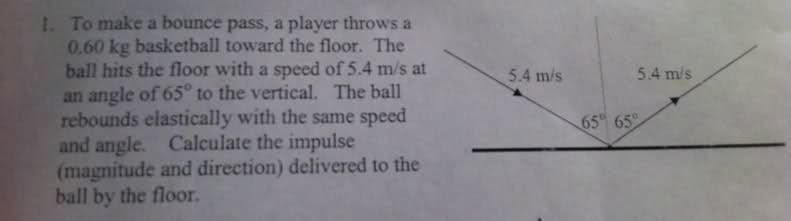# Calculating the Impulse (Magnitude and direction) question - Thanks

## Homework Statement

Below is a snapshot of the question I cant seem to solve.I cant seem to find in my text book how to work out this problem...:(

Any pointers? Thanks!

berkeman
Mentor

## Homework Statement

Below is a snapshot of the question I cant seem to solve.I cant seem to find in my text book how to work out this problem...:(

Any pointers? Thanks!

## The Attempt at a Solution

What is the mathematical definition of the impulse?

Impulse is the change in momentum

berkeman
Mentor
Impulse is the change in momentum

Show us the equation(s). That would be what you should have put in the "Relevant Equations" part of the Homework Help Template above...

Then tell us what you think you should do with the equation(s). That would go in the last part of the HH Template...

Is this correct??

ΔPx = m(Vfx - Vix) = .60(-v sin 65 - v sin 65)

= -2mvsin65

-2(.60)(5.4)(.90) = - 5.8

Ok now im gettin stuck?